Mathematics
Easy

Question

# These two triangles are congruent. If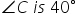, then  the measure of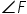is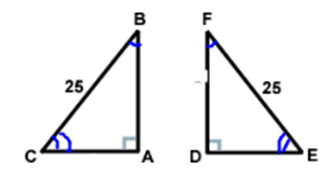## 40°50°90°45°Hint:

## The correct answer is: 50°

Mathematics

Mathematics

Mathematics

### Are these triangles congruent? If so, why?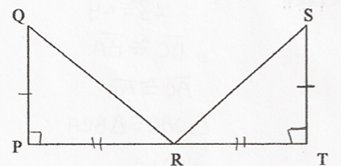Mathematics

Mathematics

Mathematics

### Can the HL congruence theorem be used to prove the triangles congruent? If so, write a congruence statement.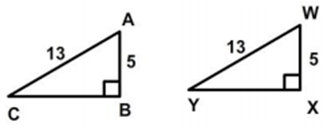Mathematics

Mathematics

Mathematics

### Complete the congruence statement: ∆ BCA =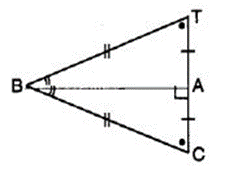Congruent triangles are triangles that have same size and shape. This suggests us that the corresponding sides are equal and corresponding angles are equal.
When two triangles are congruent, all of their corresponding sides and angles are equal.

### Complete the congruence statement: ∆ BCA =Congruent triangles are triangles that have same size and shape. This suggests us that the corresponding sides are equal and corresponding angles are equal.
When two triangles are congruent, all of their corresponding sides and angles are equal.Mathematics

### We want to show that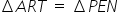. We have to use SAS criterion. We have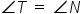, RT = EN, then____________.

SAS congruence is the term which is also known as Side Angle Side congruence. In this, triangles are congruent if any pair of corresponding sides and their included angles are equal in both triangles.

### We want to show that. We have to use SAS criterion. We have, RT = EN, then____________.

SAS congruence is the term which is also known as Side Angle Side congruence. In this, triangles are congruent if any pair of corresponding sides and their included angles are equal in both triangles.

Mathematics

Mathematics

### If ∆ ABC ≅ ∆ PQR, then the value of ∠A will be,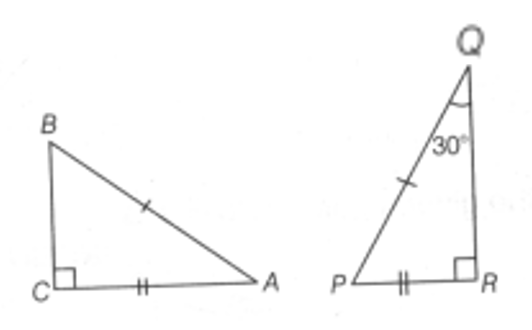Mathematics

### ∆ PQR is congruent to ∆ STU (in figure), then length of TU is ___________.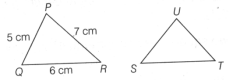Congruent triangles are triangles that have same size and shape. This suggests us that the corresponding sides are equal and corresponding angles are equal.
When two triangles are congruent, all of their corresponding sides and angles are equal.

### ∆ PQR is congruent to ∆ STU (in figure), then length of TU is ___________.Congruent triangles are triangles that have same size and shape. This suggests us that the corresponding sides are equal and corresponding angles are equal.
When two triangles are congruent, all of their corresponding sides and angles are equal.

Mathematics

Mathematics

### If ∆ ABC and ∆ DBC are on the same base BC, AB = DC and AC= DB, then __________gives a congruence relationship.#### With Turito Foundation.#### Get an Expert Advice From Turito.